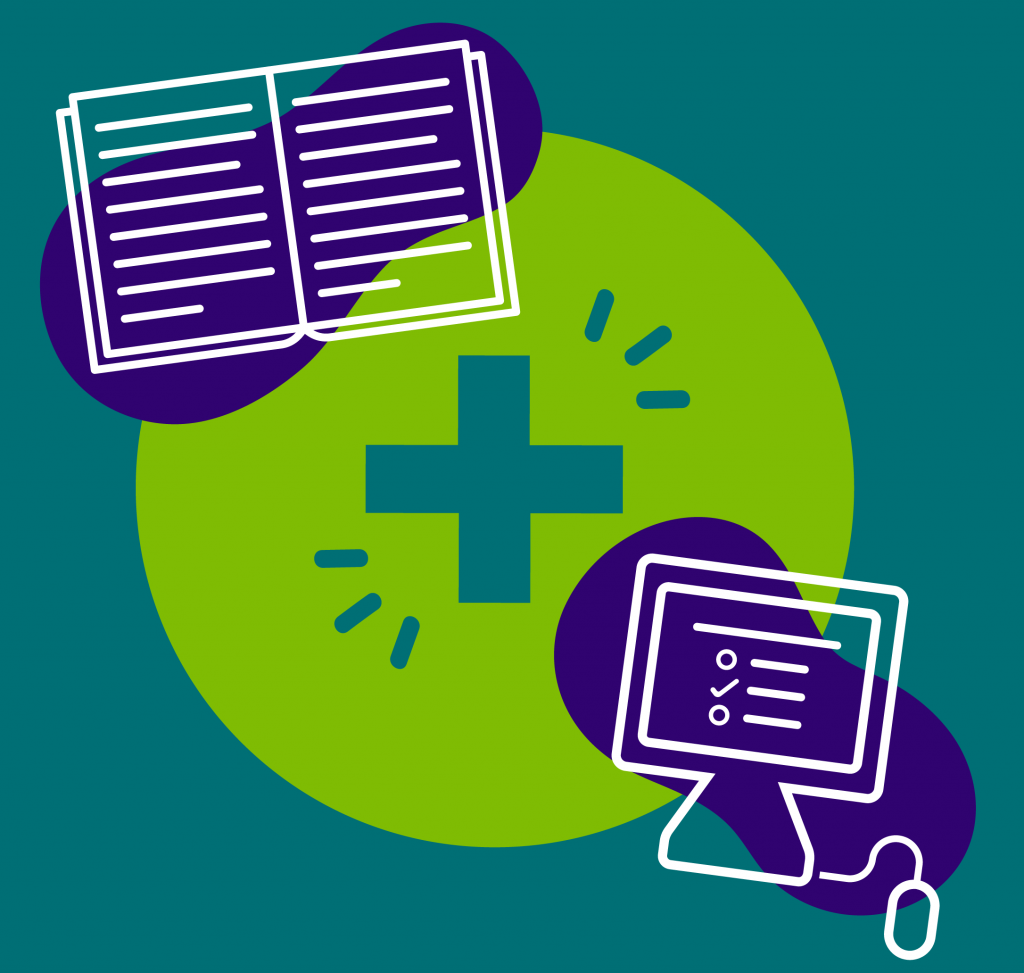###4th grade students are now a part of our Blended Learning program, a combination of traditional pencil and paper, along with activities online.

A Grade Ahead’s 4th grade Enrichment at Home math worksheets begin preparing students with a foundation of skills necessary to excel in advanced math. Students in our Enrichment at Home program apply all operations in varying formats, including word problems. Concepts students learn from our 4th grade enrichment curriculum include, but are not limited to:

• Test-taking skills and methods
• Metric measurements
• Probability and statistics
• Algebra and equations
• Geometry

#### Don’t forget, your child can begin the curriculum at any time throughout the year. Start learning today!

You may also like 4th Grade English.

Get a sample instantly!
• ## Curious about our material? Get a sample instantly!

• This field is for validation purposes and should be left unchanged.

June
Month 1
Curriculum Topics
Place value; Rounding; Estimation
Numerical Path
Multiply/Divide/Fractions 1:
Examples: 1,569 ÷ 4 = ? and 3/12 = 1/4
July
Month 2
Curriculum Topics
Factors & multiplication; Algebra
Numerical Path
Multiply/Divide/Fractions 2:
Examples: 457 x 45 = ? and 6/27 = 2/9
August
Month 3
Curriculum Topics
Time; Graphs
Numerical Path
Multiply/Divide/Fractions 3:
Examples: 256 x 15 = ? and 8/10 = x/5
September
Month 4
Curriculum Topics
PEMDAS; 2-Step word problems; Metric system
Numerical Path
Multiply/Divide/Fractions 4:
Examples: 12,030 ÷ 19 and 5/12 < 2/4
October
Month 5
Curriculum Topics
Length; Weight; Liquid volume
Numerical Path
Algebraic Thinking 2:
Examples: 22 x _ = 66 and _ ÷ 5 = 12
November
Month 6
Curriculum Topics
Geometry
Numerical Path
Fractions 1:
Examples: 27/6 = 4 1/2 and 1 4/8 = 3/2
December
Month 7
Curriculum Topics
Geometry; Fractions
Numerical Path
Decimals 1:
Example: 24.65 + 37.52 and 75.29 - 49.82
January
Month 8
Curriculum Topics
Decimals; Money
Numerical Path
Decimals 2:
Examples: 221.5 rounds to 222.0 and 13.67 rounds to 13.70
February
Test Prep Month
Curriculum Topics
Standardized Test Preparation
Numerical Path
Decimals 3:
Examples: 79.205 rounds to 79.210 and 140.97 rounds to 141.0
March
Month 9
Curriculum Topics
Algebra; Coordinate grid; Statistics
Numerical Path
Fractions/Decimals 1:
Examples: 39/10 -> 3.9 and 0.2 -> 1/5
April
Month 10
Curriculum Topics
Graphical data; Probability; Properties
Numerical Path
Fractions/Decimals 2:
Examples: 3/8 -> 0.375 and 3.9 -> 3 9/10
May
Month 11
Curriculum Topics